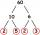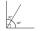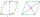# Given

Given 2x =0.125 find the value of x

x =  0.0625

### Step-by-step explanation:

2x=0.125

2•x=0.125

2x = 0.125

x = 1/16 = 0.0625

Our simple equation calculator calculates it.Did you find an error or inaccuracy? Feel free to write us. Thank you!Tips to related online calculators
Do you have a linear equation or system of equations and looking for its solution? Or do you have a quadratic equation?

## Related math problems and questions:

• Find unknown 2Find unknown denominator: 2/3 -5/? = 1/4
• Value of expressionX=2, y=-5 and z=3 what is the value of x-2y?
• Evaluate expr with 2 varsIf a=6 and x=2, find the value of (2ax + 7x- 10) /(4ax-3a-2)
• Midpoint 5FM=3x-4, MG=5x-26, FG=? Point M is the midpoint of FG. Use the given information to find the missing measure or value.
• Simple equation 324 = n • 27, solve for n
• ValueDetermine the value of the following exspressions: a) (23-25)·(4-5) b) (97-123):(18+8)
• Complementary angles 2Two complementary angles are (x+4) and (2x - 7) find the value of x
• Evaluate expressionEvaluate following expression: 5 to the power of 2 - (4x4) divided by 2 =
• A number 5A number is divisible by 24, 25, and 120 if it is increased by 20. Find the number.
• Two thirdsFind two-thirds of the number equal to two-thirds of 99
• Six paintersSix painters paint an area of 120 m2 in 4 hours. How long does it take for 12 painters to paint 480 m2 with the same performance?
• The areaThe area of a rhombus is 143 m2. If the longer diagonal is 26 m, find the shorter diagonal in inches named d1.
• Fraction eq2/3x + 5/7 = 1/2x + 22/21
• Equation 29Solve next equation: 2 ( 2x + 3 ) = 8 ( 1 - x) -5 ( x -2 )Find the radius of the circle with area S = 200 cm².2x + 3 + 7x = – 24, what is the value of x?There are given numbers A = 135, B = 315. Find the smallest natural number R greater than 1 so that the proportions R:A, R:B are with the remainder 1.